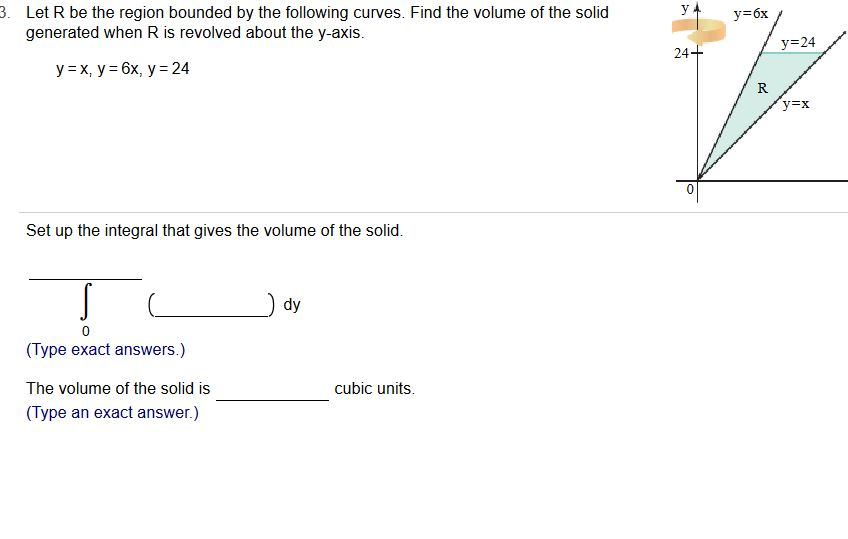# . Let R be the region bounded by the following curves. Find the volume of the solidу-бхgenerated when R is revolved about the y-axis.24R.0Set up the integral that gives the volume of the solid.dy(Type exact answers.)The volume of the solid is(Type an exact answer.)cubic units.

Question
15 viewshelp_outlineImage Transcriptionclose. Let R be the region bounded by the following curves. Find the volume of the solid у-бх generated when R is revolved about the y-axis. 24 R. 0 Set up the integral that gives the volume of the solid. dy (Type exact answers.) The volume of the solid is (Type an exact answer.) cubic units. fullscreen
check_circle

Step 1

we will calculate volume by washer metho...

### Want to see the full answer?

See Solution

#### Want to see this answer and more?

Solutions are written by subject experts who are available 24/7. Questions are typically answered within 1 hour.*

See Solution
*Response times may vary by subject and question.
Tagged in

### Integration